# Two ribbons

The total length of the two ribbons is 13 meters. If one ribbon is 7 and 5/8 meters long, what is the length of the other ribbon?

b =  5.375 m

### Step-by-step explanation: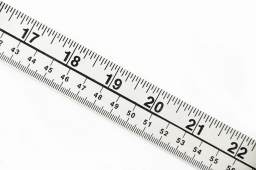Did you find an error or inaccuracy? Feel free to write us. Thank you!Tips to related online calculators
Need help to calculate sum, simplify or multiply fractions? Try our fraction calculator.
Do you want to convert length units?

## Related math problems and questions:

• KrishnaKrishna bought 5 1/2 meters of ribbon from the market . She cut off a 2 3/4 m piece of ribbon from it and gave it to Sushima . What length of ribbon is left with her?
• Dividing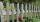Divide the three-line segment 13 cm, 26 cm, and 19.5 cm long for parts so that the individual pieces were equally long and longest. How long will the individual parts, and how many will it?Faye had a piece of ribbon. After using 3/8 meter for her headband, she had 1/4 meter left. How many meters of ribbon did she have at first?
• Divide in ratioLine segment AB 12 cm long divide in a ratio of 5: 3. How long are the individual parts?
• Map 2At what scale is made map if the distance 8.2 km corresponds on the map segment 5 cm long?
• Wood 11Father has 12 1/5 meters long wood. Then I cut the wood into two pieces. One part is 7 3/5 meters long. Calculate the length of the other wood?
• Ribbon on the cube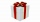A cubical gift box is tied with a piece of ribbon. If the total length of the free ends and the bow is 18 inches, what is the length of the ribbon used? (Each side of the cube is 6 inches).
• Hannah and Emily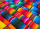Hannah and Emily need to use  4 1/4  feet of ribbon to finish an art project. Hannah has 2 3/4 feet of ribbon and Emily has 1 3/4 feet of ribbon. How much ribbon will they have left over after they finish their art project?
• KarinaKarina has 5 4/7 meters of cloth. She needs 3 1/6 meters of it in making a curtain for her window. How many meters of cloth were left to her after making the curtain?
• Points on line segmentPoints P & Q belong to segment AB. If AB=a, AP = 2PQ = 2QB, find the distance: between point A and the midpoint of the segment QB.
• SegmentsWhich of the pairs of numbers on the number line encloses the longest segment: ?
• Eight pipes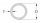Eight pipes are each 2¼m long . what is the total length of the eight pipes?
• Line segmentThe 4 cm long line segment is enlarged in the ratio of 5/2. How many centimeters will measure the new line segment?
• Fractions and mixed numerals(a) Convert the following mixed numbers to improper fractions. i. 3 5/8 ii. 7 7/6 (b) Convert the following improper fraction to mixed number. i. 13/4 ii. 78/5 (c) Simplify these fractions to their lowest terms. i. 36/42 ii. 27/45 2. evaluate following ex
• Line segment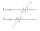Line segment AB is 8 cm long. Divide it in a ratio of 2: 3.
• Eight 9Eight pipes are 2¼ meter long . what is the total length of the eight pipes?
• Line segmentCut a line segment of 15 cm into two line segments so that their lengths are in ratio 2:1. What length will each have?